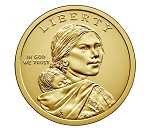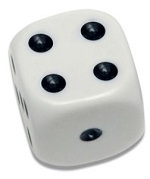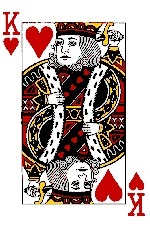# Probability

Probability is about how Likely something is to occur, or how likely something is true.

The mathematic probability is a Number between 0 and 1.

0 indicates Impossibility and 1 indicates Certainty.

## The Probability of an Event

The probability of an event is:

The number of ways the event can happen / The number of possible outcomes.

Probability = # of Ways / Outcomes

## Tossing CoinsWhen tossing a coin, there are two possible outcomes:

WayProbability
Tails1/2 = 0.5

## P(A) - The Probability

The probability of an event A is often written as P(A).

When tossing two coins, there are 4 possible outcomes:

EventP(A)
Tails + Tails1/4 = 0.25

## Throwing DicesWhen throwing a dice, there are 6 possible outcomes:

EventP(A)
Lands on 11/6 = 0.166666
Lands on 21/6 = 0.166666
Lands on 31/6 = 0.166666
Lands on 41/6 = 0.166666
Lands on 51/6 = 0.166666
Lands on 61/6 = 0.166666

The possibility of throwing 3 fours at the same time is
(1/6)3 (Lands on 4 to the power of 3):

The possibility is:

let p = Math.pow(1/6, 3);

Try it Yourself »

The possibility of throwing 3 likes at the same time is 6 times larger:
(lands on 1) + (Lands on 2) + ... + (Lands on 6)

The possibility is:

let p = Math.pow(1/6, 3) * 6;

Try it Yourself »

## 6 Balls

I have 6 balls in a bag: 3 reds, 2 are green, and 1 is blue.

Blindfolded. What is the probability that I pick a green one?

Number of Ways it can happen are 2 (there are 2 greens).

Number of Outcomes are 6 (there are 6 balls).

Probability = Ways / Outcomes

The probability that I pick a green one is 2 out of 6: 2/6 = 0.333333.

The probability is written P(green) = 0.333333.

P(A)W/OProbability
P(red)3/60.5000000
P(green)2/60.3333333
P(blue)1/60.1666666

## P(A) = P(B)

 P(A) = P(B) Event A and B have the same chance to occur P(A) > P(B) Event A has a higher chance to occur P(A) < P(B) Event A has a lower chance to occur

For the 6 balls:

 P(red) > P(green) I am more likely to pick a red than a green P(red) > P(blue) I am more likely to pick a red than a blue P(green) > P(blue) I am more likely to pick a green than a blue P(blue) < P(green) I am less likely to pick a blue than a green P(blue) < P(red) I am less likely to pick a blue than a red P(green) < P(red) I am less likely to pick a green than a red

## Choosing a KingThe probability of choosing a king in a deck of cards is 4 in 52.

Number of Ways it can happen are 4 (there are 4 kings).

Number of Outcomes are 52 (there are 52 cards).

Probability = Ways / Outcomes

The probability is 4 out of 52: 4/52 = 0.076923.

The probability is written P(king) = 0.076923.

W3Schools is optimized for learning and training. Examples might be simplified to improve reading and learning. Tutorials, references, and examples are constantly reviewed to avoid errors, but we cannot warrant full correctness of all content. While using W3Schools, you agree to have read and accepted our terms of use, cookie and privacy policy.